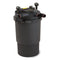Your pond filter is important to maintaining a healthy ecosystem in your pond. A properly sized filter keeps your water clear and makes for happy fish, plants and beneficial bacteria.

1. To correctly size a pond filter, you will need to know the volume - how many gallons of water are in your pond. If you don't already have this calculation, you can use the formulas below (formulas are based on measurements in feet and inches).

2. For a rectangular or square pond: length x width x depth x 7.5 = gallons

3. For an oval pond with straight sides: length x width x depth x 6.7 = gallons

4. For a circular pond: diameter x diameter x depth x 5.9 = gallons

5. Now that you've calculated the number of gallons, sizing the filter is easy. The rule of thumb is that you should have a filter that is rated for about 1.5 times the number of gallons in your pond. So if you calculated your pond contains 400 gallons, 1.5 x 400 would mean a filter rated at 600 gallons per hour (gph). All pond filters will have a flow rate measured in gph.

If you have any other questions about pool and spa products please do let us know - we are here to help!